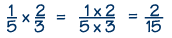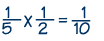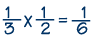Home    |    Teacher    |    Parents    |    Glossary    |    About UsTo multiply fractions, first we simplify the fractions if they are not in lowest terms. Then we multiply the numerators of the fractions to get the new numerator, and multiply the denominators of the fractions to get the new denominator. Simplify the resulting fraction if possible.

Note that multiplying fractions is frequently expressed using the word "of." For example, to find one-fifth of 10 pieces of candy, you would multiply 1/5 times 10, which equals 2. Study the example problems to see how to apply the rules for multiplying fractions.

Example 1Example 2Example 3Homework Help | Pre-Algebra | FractionsEmail this page to a friendSearch·  Definitions·  Reducing fractions·  Adding and subtract-     ing fractions·  Multiplying fractions·  Dividing fractions·  Adding and subtract-     ing mixed numbers·  Multiplying     mixed numbers·  Dividing     mixed numbersFirst Glance In Depth Examples WorkoutMultiplying fractions